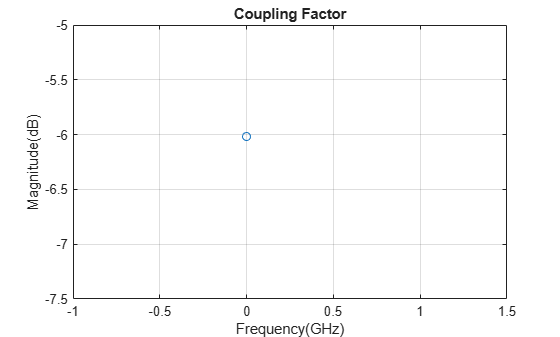# coupling

Calculate coupling factor of coupler

Since R2022a

## Syntax

``coupling(coupler,frequency)``
``c = coupling(coupler,frequency)``

## Description

example

````coupling(coupler,frequency)` calculates and plots the coupling factor of a coupler over the specified frequency values.```

example

````c = coupling(coupler,frequency)` returns the coupling factor of a coupler over the specified frequency.```

## Examples

collapse all

Create a branchline coupler with default values.

`coupler = couplerBranchline`
```coupler = couplerBranchline with properties: PortLineLength: 0.0186 PortLineWidth: 0.0051 SeriesArmLength: 0.0184 SeriesArmWidth: 0.0083 ShuntArmLength: 0.0186 ShuntArmWidth: 0.0051 Height: 0.0016 GroundPlaneWidth: 0.0600 Substrate: [1x1 dielectric] Conductor: [1x1 metal] IsShielded: 0 ```

Calculate the coupling factor of the coupler at 2 GHz.

`coupling(coupler,2e6)`Create a rat-race coupler with default values.

`coupler = couplerRatrace;`

Calculate the coupling factor of the coupler at 3 GHz.

`c = coupling(coupler,3e6)`
```c = -6.0206 ```

## Input Arguments

collapse all

Coupler, specified as a coupler object. For a complete list of couplers, see Splitters and Couplers.

Frequency to calculate the coupling, specified as an integer in Hz or as a vector with each element specified in Hz.

## Version History

Introduced in R2022a